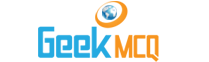# CSS :: Market Equilibrium

1.  A decrease in demand causes the equilibrium price to:
 A. Rise B. Fall C. Remain constant D. Indeterminate

2.  When price is below equilibrium level, there will be:
 A. Surplus commodity in the market B. Shortage of commodity in the market C. Supply curve will shift D. Demand curve will shift

3.  If equilibrium price rises but equilibrium quantity remains unchanged, the cause is:
 A. Supply and demand both increase equally B. Supply and demand both decrease equally C. Supply decreases and demand increases D. Supply increases and demand decreases

4.  Price of a product is determined in a free market:
 A. By demand for the product B. By supply of the product C. By both demand and supply D. By the government

5.  An increases in the price of mutton provides information which:
 A. Tells consumers to buy more mutton B. Tells consumers to buy more chicken C. Tells producers to produce more mutton D. Provides no information

6.  In market equilibrium, supply is vertical line. The downward sloping demand curve shifts to the rights. Then:
 A. Price will fall B. Price remains same C. Price will rise D. Quantity rises

7.  Equilibrium:
 A. Is a state that can never be achieved in economics B. Is an important idea for predicting economic changes C. Is a stable condition D. Is an unstable condition

8.  Ten rupees is the equilibrium price for good X. If government fixes price at Rs. 5, there is:
 A. A shortage B. A surplus C. Excess supply D. Loss

9.  A rise in supply and demand in equal proportion will result in:
 A. Increase in equilibrium price and decrease in equilibrium quantity B. Decrease in equilibrium price and increase in equilibrium quantity C. No change in equilibrium price and increase in equilibrium quantity D. Increase in equilibrium price and no change in equilibrium quantity

10.  The price and sales of sugar both increase. What could be the cause of this?
 A. A decrease in the incomes of the consumers B. A decrease in the tax on sugar C. An increase in the wages of workers in the sugar industry D. An increase in the price of sugar substitutes